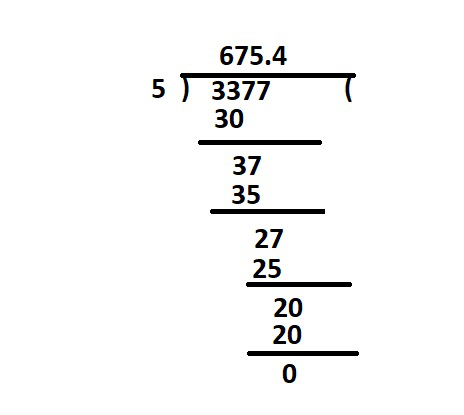# How to Divide two Decimals?

• Last Updated : 16 May, 2022

Dividing decimals is similar to dividing whole numbers, except that the position of the decimal point must be remembered. When we divide decimals, We convert the divisor into a whole number by pushing the decimal point all the way to the right. The dividend’s decimal point is then moved up to the same number of places to the right, and the resulting numbers are divided in the same manner as for regular division.

### Decimals

Decimals are used in the extended number system to express tenths, hundredths, and thousandths, along with other factors. Working with decimals may appear to be more difficult, yet the many procedures involving decimal numbers are quite similar to those using whole numbers. A decimal number consists of a whole number and a fractional component separated by a dot. For example,

34.15 is a decimal number containing 34 in the numerical component and 15 in the decimal part.

This may be explained as follows,

34.15 = 30 + 4 + 0.1 + 0.05.

### How to Divide two Decimals?

Solution:

To divide two decimals we have to understand this in details, i.e; Both the dividend and the divisor in this division are decimals, and we may solve them using one of two ways. In the first method, we first move the divisor’s decimals to make it a whole number, and then we move the dividend’s decimals.

Step 1: To divide 24.5 by 3.5, for example, move the divisor’s decimal to the right as many times as necessary to make it a whole number. This results make the divisor be 35.

Step 2: Move the decimal in the dividend by the same amount of digits you moved it in the divisor. This results in the divisor 245 .

Step 3: Divide the dividend (245) by the divisor (35) as you normally would.

And at last will give the result of dividing 245 ÷ 35 = 7. Second Method, when the number of digits after the decimal in the dividend is greater or less than the number of decimals in the divisor, the second method is used.

First case, If the decimal place of the dividend is higher than or equal to the decimal place of the divisor:

Step 1: Ignore the decimal and divide normally till the remaining is 0.

For example: To divide: 0.009 ÷ 0.3, , we ignore the decimals and express it as 9 ÷ 3 = 3.

Step 2: Use the following formula to place the decimal point,

Dividend’s decimal place – Divisor’s decimal place = Quotient’s decimal place.

Using the formula, we now get 3 -1 = 2. As a result, the decimal of the quotient will be 0.03.

Second case, If the decimal place of the dividend is smaller than the decimal place of the divisor: Convert the decimal to a fraction in this example.

For instance:  0 .5÷ 0.02

= 5/10 ÷ 2/100

= 5/10 × 100/2

= 25

So this way we can divide two decimals.

### Sample Questions

Question 1: Find the quotient for 1.564 ÷ 0.013.

Solution:

The given Divisor = 0.013 and Dividend = 1.564

Now, change the divisor 0.013 to a whole number by moving the decimal point 3 places to the right. Then move the decimal point in the dividend 1.564 the same, 3 places to the right.

So, 1.564 ÷ 0.013

= 1564 ÷ 13

By simplifying we will get 120.30 as quotient.

Question 2: Simplify  if 0.6 ÷ 0.02.

Solution:

Here the decimal place of the dividend is smaller than the decimal place of the divisor:

Convert the decimal to a fraction

So here,

= 6/10 ÷ 2/100

= 6/10 × 100/2

= 600/20

= 30

Question 3: Simplify 0.0012 ÷ 0.3.

Solution:

Here the decimal place of the dividend is higher than the decimal place of the divisor.

Step 1: Ignore the decimal and divide normally till the remaining is 0.

To divide: 0.0012 ÷ 0.3, , we ignore the decimals and express it as 12 ÷ 3 = 4

Step 2: Use the following formula to place the decimal point :

Dividend’s decimal place – Divisor’s decimal place = Quotient’s decimal place.

Using the formula, we now get 4 -1 = 3 .

As a result, the decimal of the quotient will be 0.004.

Question 4: Divide 337.70 by 0.5?

Solution:

We first move the divisor’s decimals to make it a whole number, and then we move the dividend’s decimals.

Step 1: To divide 337.70 by 0.5,

First move the divisor’s decimal to the right as many times as necessary to make it a whole number. This results make the divisor be 5.

Step 2: Move the decimal in the dividend by the same amount of digits you moved it in the divisor. This results in the divisor 3377.0 or 3377.

Step 3: Divide the dividend (3377) by the divisor (5) as you normally would.dividation

At last will give the result of dividing 3377 ÷ 5 = 675.4

Question 5: Simplify  if 0.125 ÷ 0.0005

Solution:

Here the decimal place of the dividend is smaller than the decimal place of the divisor:

Convert the decimal to a fraction

So here,

= 0 .125 ÷ 0.0005

= 125/1000 ÷ 5/10000

= (125 × 10000 )/ (5 × 1000)

= 250

Question 6: Calculate: 9.91 ÷ 3.3.

Solution:

The given Divisor = 3.3  and Dividend = 9.91.

Now, change the divisor 3.3 to a whole number by moving the decimal point 1 place to the right. Then move the decimal point in the dividend 9.91 the same, 1 place to the right.

=  991 ÷ 33

= 3.003

My Personal Notes arrow_drop_up
Recommended Articles
Page :